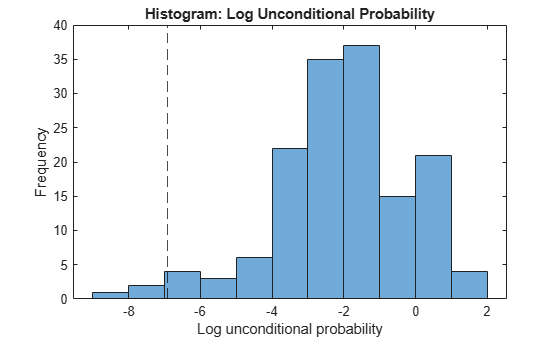# logp

Log unconditional probability density for naive Bayes classifier

## Syntax

``lp = logp(Mdl,tbl)``
``lp = logp(Mdl,X)``

## Description

``lp = logp(Mdl,tbl)` returns the log Unconditional Probability Density (`lp`) of the observations (rows) in `tbl` using the naive Bayes model `Mdl`. You can use `lp` to identify outliers in the training data.`

example

``lp = logp(Mdl,X)` returns the log unconditional probability density of the observations (rows) in `X` using the naive Bayes model `Mdl`.`

## Examples

collapse all

Compute the unconditional probability densities of the in-sample observations of a naive Bayes classifier model.

Load the `fisheriris` data set. Create `X` as a numeric matrix that contains four petal measurements for 150 irises. Create `Y` as a cell array of character vectors that contains the corresponding iris species.

```load fisheriris X = meas; Y = species;```

Train a naive Bayes classifier using the predictors `X` and class labels `Y`. A recommended practice is to specify the class names. `fitcnb` assumes that each predictor is conditionally and normally distributed.

`Mdl = fitcnb(X,Y,'ClassNames',{'setosa','versicolor','virginica'})`
```Mdl = ClassificationNaiveBayes ResponseName: 'Y' CategoricalPredictors: [] ClassNames: {'setosa' 'versicolor' 'virginica'} ScoreTransform: 'none' NumObservations: 150 DistributionNames: {'normal' 'normal' 'normal' 'normal'} DistributionParameters: {3x4 cell} Properties, Methods ```

`Mdl` is a trained `ClassificationNaiveBayes` classifier.

Compute the unconditional probability densities of the in-sample observations.

`lp = logp(Mdl,X);`

Identify indices of observations that have very small or very large log unconditional probabilities (`ind`). Display lower (`L`) and upper (`U`) thresholds used by the outlier detection method.

```[TF,L,U] = isoutlier(lp); L```
```L = -6.9222 ```
`U`
```U = 3.0323 ```
`ind = find(TF)`
```ind = 4×1 61 118 119 132 ```

Display the values of the outlier unconditional probability densities.

`lp(ind)`
```ans = 4×1 -7.8995 -8.4765 -6.9854 -7.8969 ```

All the outliers are smaller than the lower outlier detection threshold.

Plot the unconditional probability densities.

```histogram(lp) hold on xline(L,'k--') hold off xlabel('Log unconditional probability') ylabel('Frequency') title('Histogram: Log Unconditional Probability')```## Input Arguments

collapse all

Naive Bayes classification model, specified as a `ClassificationNaiveBayes` model object or `CompactClassificationNaiveBayes` model object returned by `fitcnb` or `compact`, respectively.

Sample data used to train the model, specified as a table. Each row of `tbl` corresponds to one observation, and each column corresponds to one predictor variable. `tbl` must contain all the predictors used to train `Mdl`. Multicolumn variables and cell arrays other than cell arrays of character vectors are not allowed. Optionally, `tbl` can contain additional columns for the response variable and observation weights.

If you train `Mdl` using sample data contained in a table, then the input data for `logp` must also be in a table.

Predictor data, specified as a numeric matrix.

Each row of `X` corresponds to one observation (also known as an instance or example), and each column corresponds to one variable (also known as a feature). The variables in the columns of `X` must be the same as the variables that trained the `Mdl` classifier.

The length of `Y` and the number of rows of `X` must be equal.

Data Types: `double` | `single`

collapse all

### Unconditional Probability Density

The unconditional probability density of the predictors is the density's distribution marginalized over the classes.

In other words, the unconditional probability density is

`$P\left({X}_{1},..,{X}_{P}\right)=\sum _{k=1}^{K}P\left({X}_{1},..,{X}_{P},Y=k\right)=\sum _{k=1}^{K}P\left({X}_{1},..,{X}_{P}|y=k\right)\pi \left(Y=k\right),$`

where π(Y = k) is the class prior probability. The conditional distribution of the data given the class (P(X1,..,XP|y = k)) and the class prior probability distributions are training options (that is, you specify them when training the classifier).

### Prior Probability

The prior probability of a class is the assumed relative frequency with which observations from that class occur in a population.